# Tree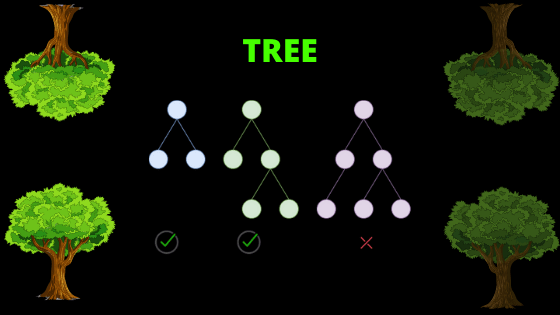## Tree

• Tree can be defined as a collection of entities (nodes) linked together to simulate a hierarchy.

## Some Terminology That Is Used In Tree

### NODE

Each data item in a tree.

### degree of node

The total numvber of childeren associated with a nodes.

### degree of a tree

Highest child of a node in a tree.

### internal node

• At least one child node.
• Internal node is also known as non-terminal node.
• Every non-leaf node is an internal node.

### Path

Sequence of consecutive edges from source node to density nodes.

### Ancestor

Any predecessor node on the path from that node to root

### decendent

Any successor node on the path from that node to leaf node

### sibling

Children of some parent

### Degree

• Degree of node is number of children of that node.
• Degree of tree = Max degree among all nodes

### Depth/level of node

Total length of path from that node to root

### Hight of node

Number of edge in the longest path from that node to a leaf

## Binary Tree

• Minimal no. of nodes in a binary tree if  height  of binary tree is H = H + 1
• if hight of binary tree is H than maximal number of nodes  H = 2H+1-1
• If level is L than no. of nodes at that lavel is 2L
• Number of nodes = 2* Internal Nodes -1
• Internal Nodes = Number of leaves -1

## Tree Traversals (Inorder, Preorder and Postorder)

Following are the commonly used methods for traversing trees.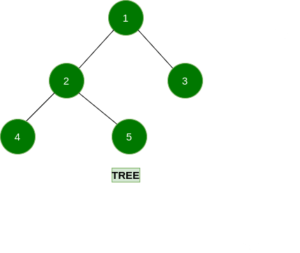Depth First Traversals:
(a)4 2 5 1 3, known as Inorder (Left, Root, Right)
(b) 1 2 4 5 3, known as Preorder (Root, Left, Right)
(c) 4 5 2 3 1, known as Postorder (Left, Right, Root) :

1 2 3 4 5: Breadth First or Level Order Traversal

## Array Representation of Binary Tree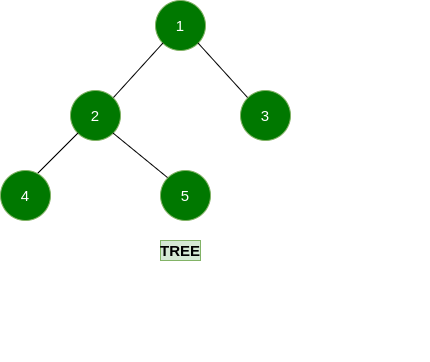##### CASE : II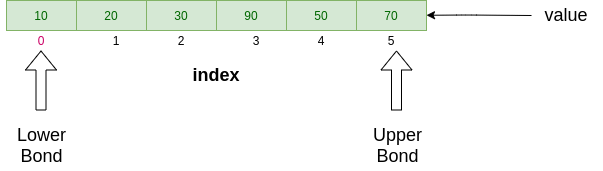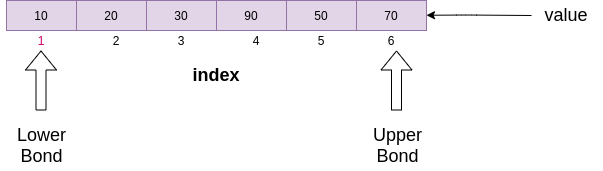FOR CASE : I

• If node is at  “i”th index :-
1. Left child = [(2*i) + 1]
2. Right child = [(2*i) +2]
3. Parent = floor[(i-1)/2]

FOR CASE : II

• If node is at “i”th index :-
1. Left child = (2*i)
2. Right child = [(2*i) + 1]
3. Parent = floor(i/2)

* Whenever you represent any binary tree into form of array the binary tree must be complete binary tree otherwise you have to first make it complete binary tree than represent in form of array.

Like Following Way

First way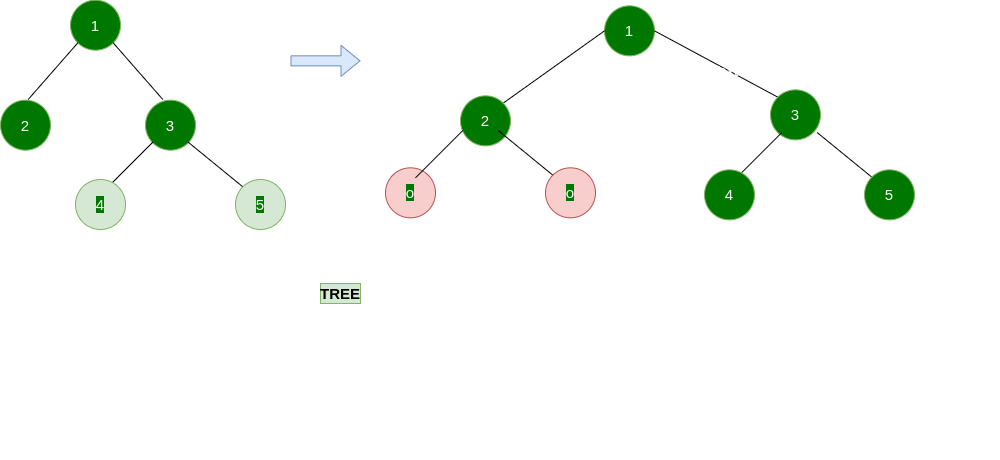Second way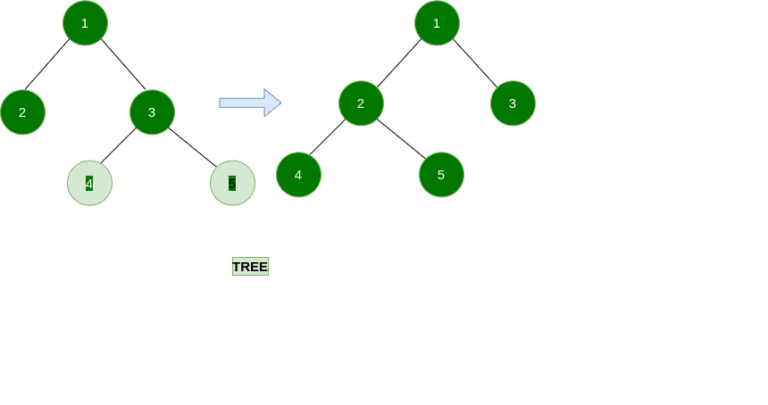## Full Binary Tree

• It is also known as Proper Binary Tree or Strict Binary Tree
• Every node have at least 0 or 2 children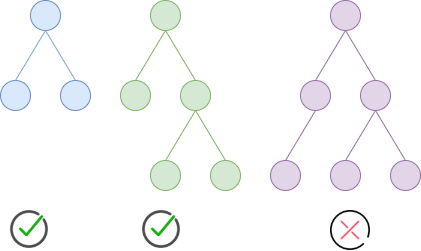## Complete Binary Tree

• All levels are totally filled (aside from perhaps the last level) and the last level has node must be as left as able to be done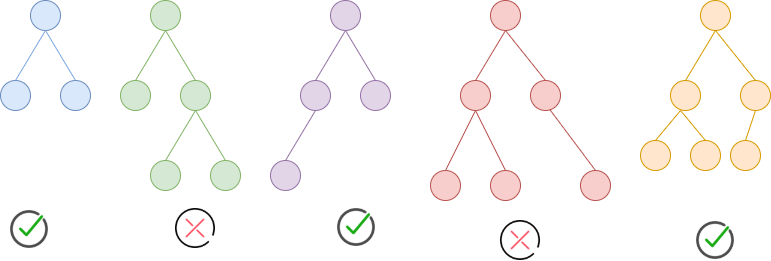## Perfect Binary Tree

• All perfect binary trees are complete or full binary tree but vice versa is not true.
• Each  internal nodes have two children and every leaf are at the same degree or level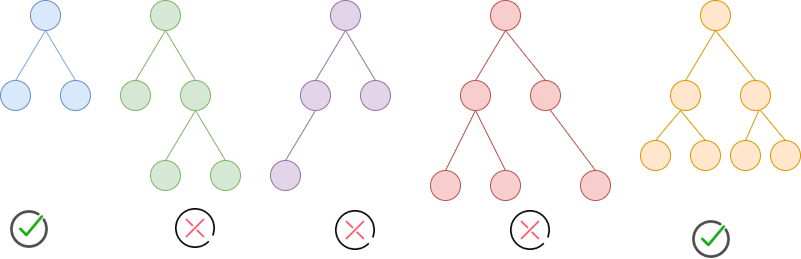## Degenerate Binary tree

• All internal nodes have one and only one child
• eg. left skewed or right-skewed tree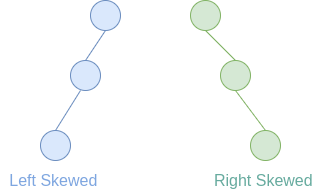## Binary Search Tree

The  maximum height of a binary search tree will be when the tree if fully skewed.

• Max height  = N-1
• Full complete tree it also represents the minimum hight of that tree
• Minimum height of a binary search tree = Log2(N+1) – 1
• Maximum number of nodes = 2H+1-1

Value(left node) < Value(root node) < Value (right node)

* N = numbers of nodes

*H = Height or edge of a tree

## AVL Tree

• if Hight is H than Maximal nodes in an AVL Tree is H = 2H+1-1
• Minimum height of an AVL Tree using N nodes = Floor (log2N)
• Minimum number of nodes in an AVL Tree of height H is given by a recursive relation : N(H) = N (H-1) + N(H-2) + 1
• Maximum height of an AVL Tree using N nodes is calculated using recursive relation : N(H) = N(H-1) + N(H-2) + 1
• Balance Factor = Left Sub Tree – Right Sub Tree i;e; [1,0,-1]
• AVL Tree all operations (Insert, delete and search) will take O(log n) time.

## B-tree

• Balanced m-way tree, where m represent the order of that tree
• B-tree is a generalization of binary search tree, In which a node can have more than one key and more than two children
• All leaf node must be at the same level

#### B-tree order-m has following properties

• Every node has maximum ‘m‘ children
• Minimum children :
• leaf -> 0
• root -> 2
• internal nodes -> ceiling (m/2)
• Every node has a maximum (m-1) key
• Minimum key :
• root node -> 1
• all others nodes -> ceiling [(m/2)-1]